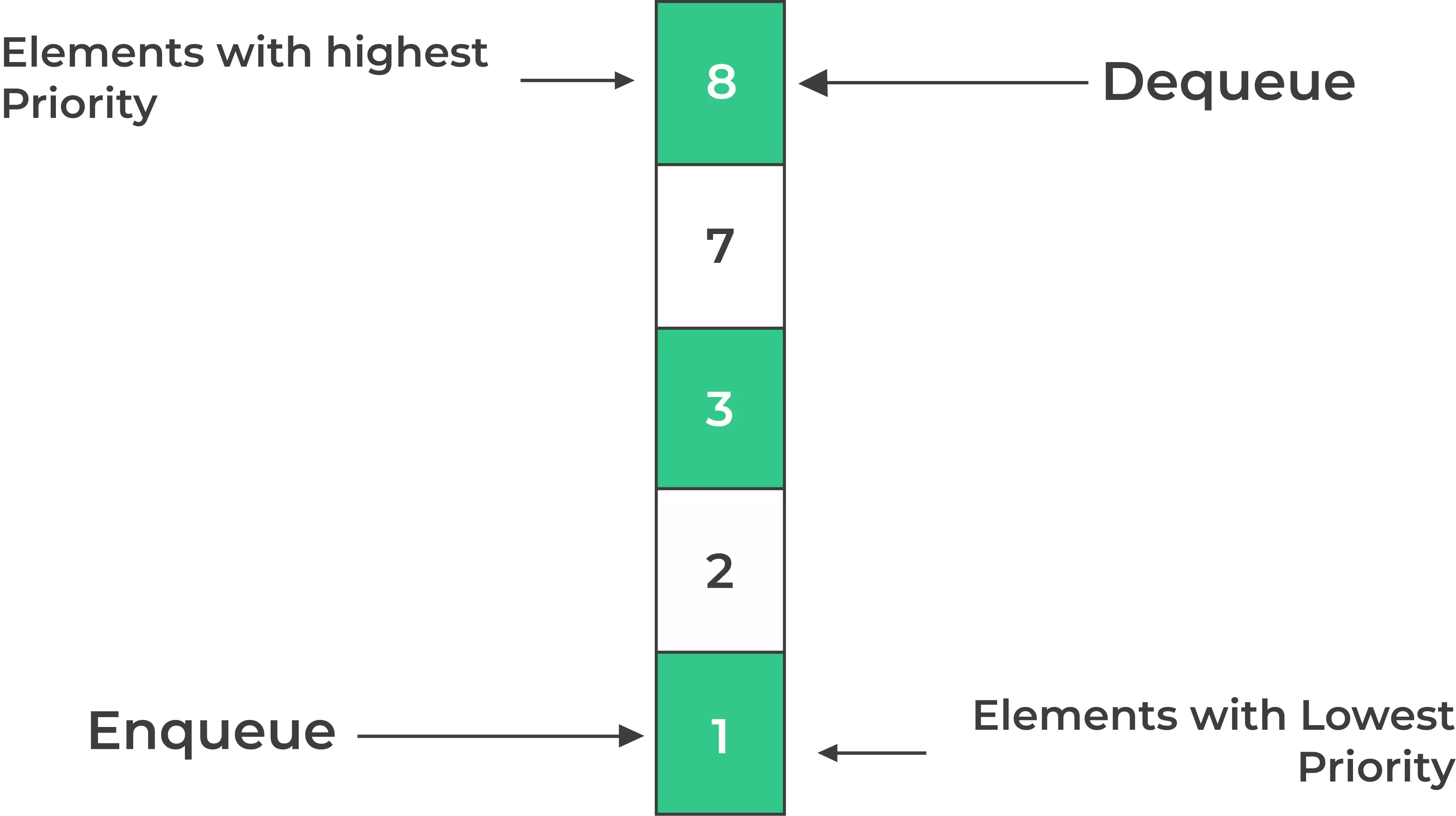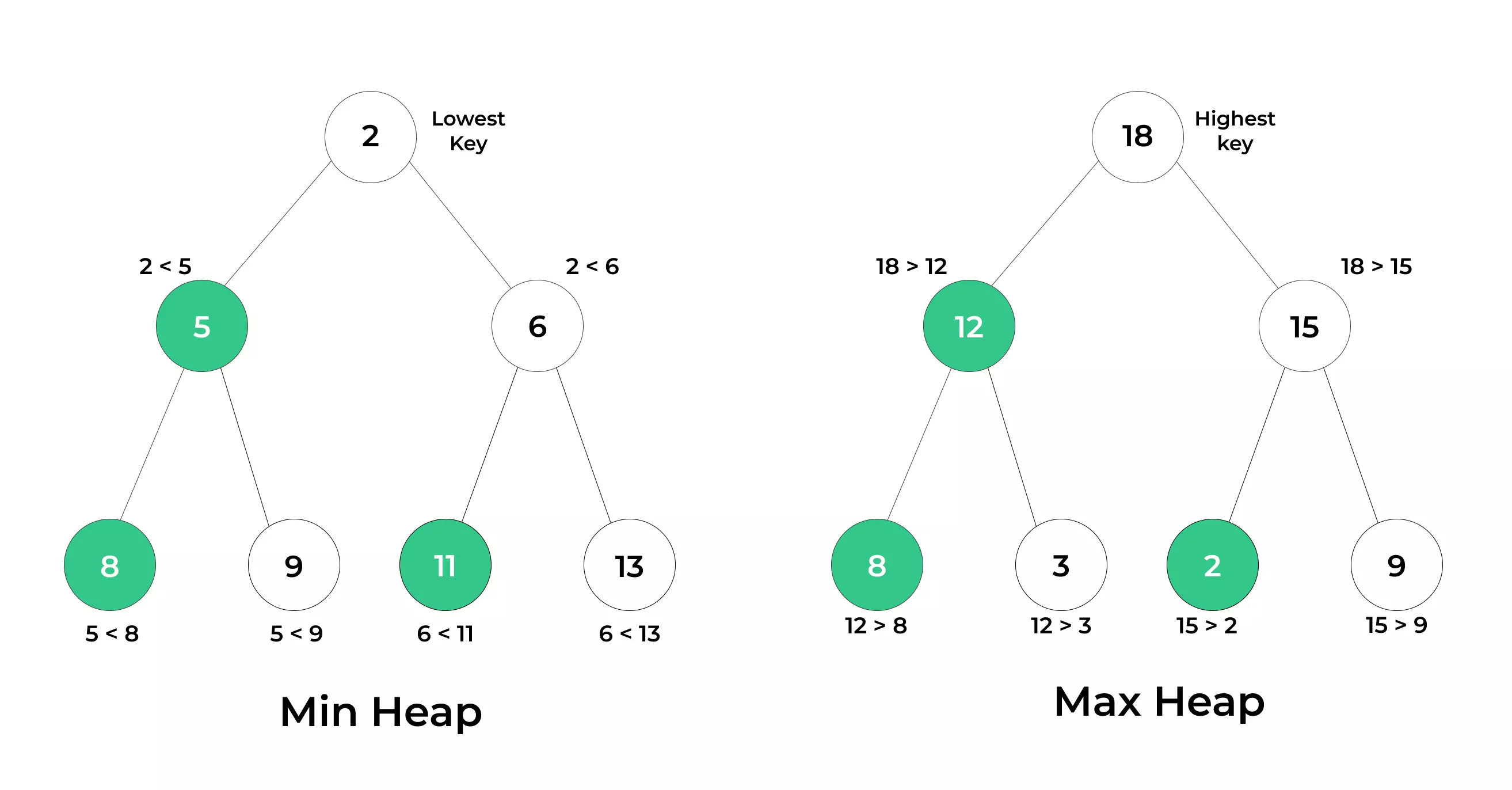# Introduction to Priority Queues using Python## Introduction to Priority Queues using Python

Introduction to Priority Queues using Python enable us to perform various operations like insertion, deletion, and retrieval of data with optimal time complexity.

In this article, we’ll delve into the concept of Priority Queues, understand their significance, and explore how to implement them using Python.

## Priority Queue Python

A Priority Queue is a type of data structure that stores elements with associated priorities. Unlike traditional queues, where elements are retrieved based on the order of insertion (FIFO),

• Priority Queues serve elements based on their priority values. Elements with higher priorities are dequeued before those with lower priorities.## How to use Priority Queue in Python?

• Import the heapq module:
```import heapq
```
• Create an empty list to represent your priority queue. You can use this list to store elements along with their priorities:
```priority_queue = []
```
• Add elements to the priority queue using the heapq.heappush() function. Each element should be a tuple where the first element is the priority and the second element is the data:
```heapq.heappush(priority_queue, (3, 'Task 1'))
```
• To retrieve the element with the highest priority, you can use the heapq.heappop() function:
```highest_priority_task = heapq.heappop(priority_queue)
```
• You can continue adding elements and popping elements from the priority queue as needed.
• If you want to check if the priority queue is empty, you can use the len() function:
```if len(priority_queue) == 0:
print("Priority queue is empty")
else:
print("Priority queue is not empty")
```## How does Python priority queue sort?

• In Python, a priority queue is typically implemented using the heapq module, which provides a heap-based priority queue.
• Python’s heapq module uses a min-heap to implement a priority queue. A min-heap is a binary tree where the parent node has a smaller (or equal) value than its children.

#### Implementing Priority Queues in Python

Python offers multiple ways to implement Priority Queues. Let’s explore two common approaches:

• Using Lists

One straightforward way to create a Priority Queue in Python is by using lists. You can maintain a list of elements and sort them based on their priorities whenever necessary.

• Using Heaps

The heapq module in Python provides a convenient way to implement Priority Queues using binary heaps. Heaps ensure efficient operations, making them an ideal choice.

## What are the advantages of priority queues in Python?

In Python, you can implement a simple Priority Queue using a list and the heapq module. Here’s an example:

### Example 1: Priority Queue using Lists

```import heapq

class PriorityQueue:
def __init__(self):
self.elements = []

def push(self, item, priority):
heapq.heappush(self.elements, (priority, item))

def pop(self):
if self.is_empty():
raise IndexError("Priority queue is empty")
return heapq.heappop(self.elements)

def is_empty(self):
return len(self.elements) == 0

# Example usage:
pq = PriorityQueue()

while not pq.is_empty():
print(pq.pop())

```
• In this example, we use a list to store elements, and heapq.heappush and heapq.heappop to maintain the heap property based on the priorities.
##### Conclusion

In this article, we’ve introduced you to the world of Priority Queues. We’ve explored their significance, types, operations, and implementation in Python. Understanding Priority Queues can significantly enhance your ability to tackle complex problems in the realm of computer science and programming.

### Related Banners

Get PrepInsta Prime & get Access to all 200+ courses offered by PrepInsta in One Subscription

## Get over 200+ course One Subscription

Courses like AI/ML, Cloud Computing, Ethical Hacking, C, C++, Java, Python, DSA (All Languages), Competitive Coding (All Languages), TCS, Infosys, Wipro, Amazon, DBMS, SQL and others